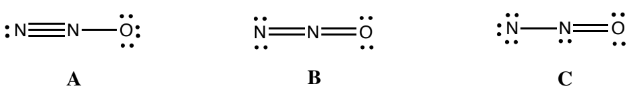# Problem: Consider the following proposed Lewis structures for N2O. Choose the best Lewis structure and calculate the formal charge on the O atom in your chosen structure.A. A is best; formal charge on O is 0.B. A is best; formal charge on O is –1.C. B is best; formal charge on O is 0.D. B is best; formal charge on O is –1.E. C is best; formal charge on O is 0.

###### FREE Expert Solution

We are asked to choose the best lewis structure for N2O.

The formal charge can be calculated using the equation below:###### Problem Details

Consider the following proposed Lewis structures for N2O. Choose the best Lewis structure and calculate the formal charge on the O atom in your chosen structure.A. A is best; formal charge on O is 0.

B. A is best; formal charge on O is –1.

C. B is best; formal charge on O is 0.

D. B is best; formal charge on O is –1.

E. C is best; formal charge on O is 0.